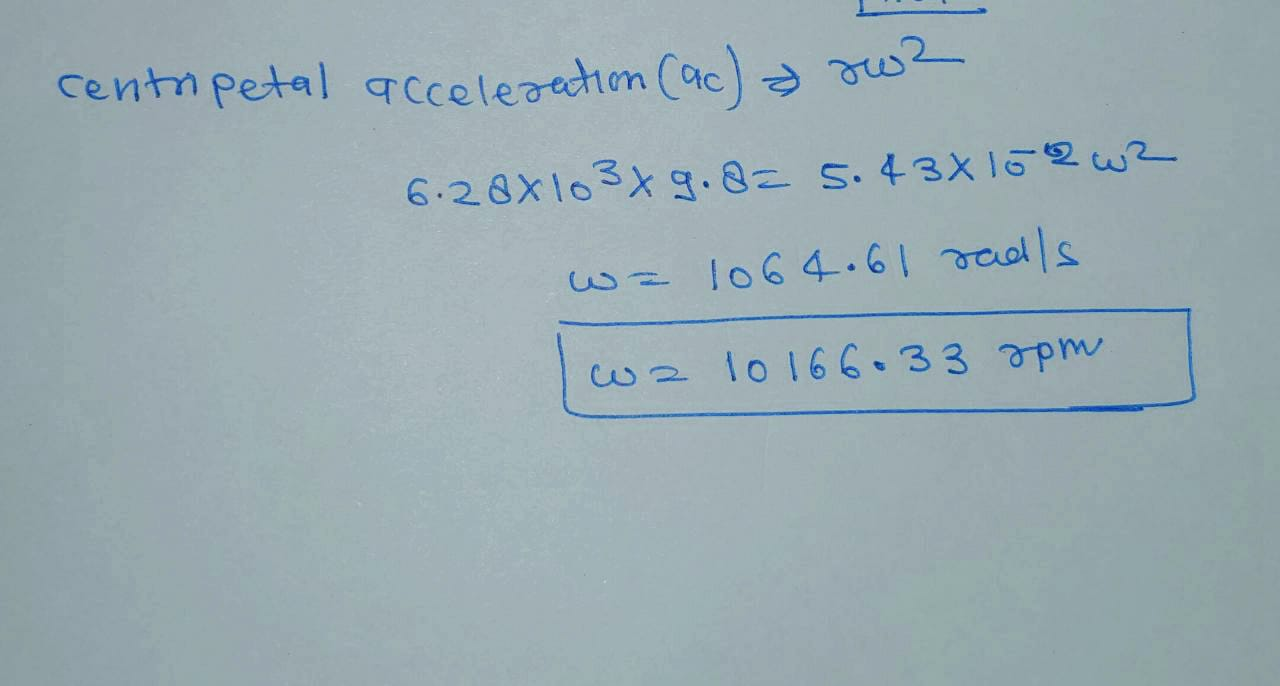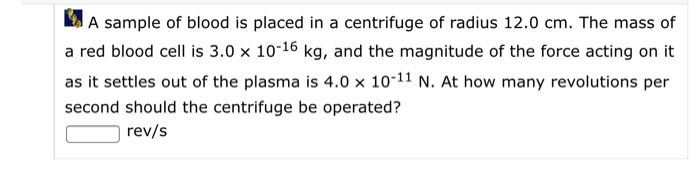Question

# A sample of blood in a centrifuge has a centripetal acceleration that is 6.28×103 times as...

1. A sample of blood in a centrifuge has a centripetal acceleration that is 6.28×103 times as large as the acceleration due to gravity. How many revolutions per minute does the sample make if it is located at a radius of 5.43cm from the axis of rotation? Do not enter unit.#### Earn Coins

Coins can be redeemed for fabulous gifts.

Similar Homework Help Questions
• ### A centrifuge is a device in which a small container of material is rotated at a...

A centrifuge is a device in which a small container of material is rotated at a high speed on a circular path. Such a device is used in medical laboratories, for instance, to cause the more dense red blood cells to settle through the less dense blood serum and collect at the bottom of the container. Suppose the centripetal acceleration of the sample is 2.61 x 103 times as large as the acceleration due to gravity. How many revolutions per...

• ### A centrifuge is a device in which small container of material is rotated at a high speed on a circular path

A centrifuge is a device in which small container of material is rotated at a high speed on a circular path. Such a device is a used in medical laboratories, for instance, to cause the more dense red blood cells to settle through the less dense blood serum and collect at the bottom of the container. Suppose the centripetal acceleration of the sample is 6.60x10^3 times as large as the acceleration due to gravity. How many revolutions per minute is...

• ### A certain centrifuge produces a centripetal acceleration of magnitude exactly 1030g at a point 11.1 cm...

A certain centrifuge produces a centripetal acceleration of magnitude exactly 1030g at a point 11.1 cm from the axis of rotation. Find the number of revolutions per second.

• ### A certain centrifuge produces a centripetal acceleration of magnitude exactly 1390g at a point 13.1 cm...

A certain centrifuge produces a centripetal acceleration of magnitude exactly 1390g at a point 13.1 cm from the axis of rotation. Find the number of revolutions per second.

• ### Steve was subjected to accelerations in a horizontal "centrifuge". It is considered that such a device...

Steve was subjected to accelerations in a horizontal "centrifuge". It is considered that such a device has a radius of 5.0 m and can generate a centripetal acceleration equivalent to 7 times the average gravitational acceleration on Earth. a) For this maximum acceleration, what is the modulus of the astronaut's speed along the circular path? b) At how many revolutions per minute should the rotation occur to achieve such acceleration? c) What is the period of this rotation?

• ### A sample of blood is placed in a centrifuge of radius 12.0 cm. The mass of a red blood cell is 3.0 ✕ 10-16 kg, and the m...A sample of blood is placed in a centrifuge of radius 12.0 cm. The mass of a red blood cell is 3.0 ✕ 10-16 kg, and the magnitude of the force acting on it as it settles out of the plasma is 4.0 ✕10-11 N. At how many revolutions per second should the centrifuge be operated? rev/s A sample of blood is placed in a centrifuge of radius 12.0 cm. The mass of a red blood cell is 3.0 x...

• ### A sample of blood is placed in a centrifuge of radius 12.0 cm. The mass of...

A sample of blood is placed in a centrifuge of radius 12.0 cm. The mass of a red blood cell is 3.0 ✕ 10-16 kg, and the magnitude of the force acting on it as it settles out of the plasma is 4.0 ✕ 10-11 N. At how many revolutions per second should the centrifuge be operated?

• ### A sample of blood is placed in a centrifuge of radius 14.0 cm. The mass of...

A sample of blood is placed in a centrifuge of radius 14.0 cm. The mass of a red blood cell is 3.0 ✕ 10-16 kg, and the magnitude of the force acting on it as it settles out of the plasma is 4.0 ✕ 10-11 N. At how many revolutions per second should the centrifuge be operated? ______rev/s

• ### A centrifuge takes 100.0s to spin up from rest to it's final angular speed with constant...

A centrifuge takes 100.0s to spin up from rest to it's final angular speed with constant acceleration. A point is located 10.0cm from the axis of rotation of the centrifuge moves with a speed of 250.0m/s when the centrifuge is at full speed. (a) What is the final angular speed? (b) What is the average angular acceleration of the centrifuge as it spins up? (c) How many revolutions does the centrifuge make as it goes from rest to it's final...

• ### A biker runs at constant speed 34 m/s around a circular track with a centripetal acceleration...

A biker runs at constant speed 34 m/s around a circular track with a centripetal acceleration of magnitude 12.5 m/s^2 a) what is track's radius ? b) what is the magnitude of angular velocity of the sprinter? c) what is the magnitude of the sprinter's angular acceleration? d) how long does it take to complete one revolution this time is called "period of motion"? e) how many revolutions per minute does the sprinter make?